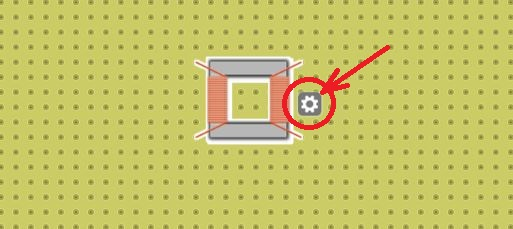# How to use Transformers

The Transformer is an static electrical energy which transfers the electrical energy from one circuit to another with the help of electromagnetic induction.

The most general use of transformer is to either increase or decrease the AC voltage. However, they too are used to isolate a part of circuit or other components.

In this post you are going to learn that how to use Transformers as per your need. Also how you can increase or decrease the voltage using the transformer in DCAClab.

## Steps to use the transformer in Lab

Step 1 : Navigate through the list of devices and components and locate the transformer as shown in the screenshot below.Step 2 : Click on the icon of the transformers as shown in the above. The transformer will appear on the board as shown in the screenshot below.Step 3 : Define the Primary as well as secondary winding and connect them as per your need.### Controlling the properties of Transformer

While working with the circuit that has a transformer, click on the Core part of the transformer. You will see a gear sign as shown in the image below.Click on the Gear sign shown as the above screenshot and a box with a label “Transformer properties” will appear as shown below.From the Transformer properties box you can control the various factors such as ratio between the two winding, their inductance and the coupling coefficient.

### Components in Transformer properties1. In this section you can set the value of inductance of the coil used in in order to make the primary as well as secondary winding. You can either use the scroll bar or the input field in order to define the value of the inductance.
2. You can also control the inductance value from the range of µ as well as mili.
3. In this you can set the ratio of number of wire turns between the two winding. For your ease of use in the DCACLAB you can follow the rule(ø) at the end of this post.
4. The Coupling coefficient (i.e. K) has an ideal condition value of “1”, however with this option you can set the coupling coefficient of the two winding to the more practical value.

Rule(ø) : Assume the Left winding as A and the right winding as B.

• The value of the ratio must be a natural number in order to make the number of winding in B bigger then A.
• The value of the ratio must be a fractional number in between 0 & 1 in order to make the number of winding in A more then B.
•
•
•
•
•
•